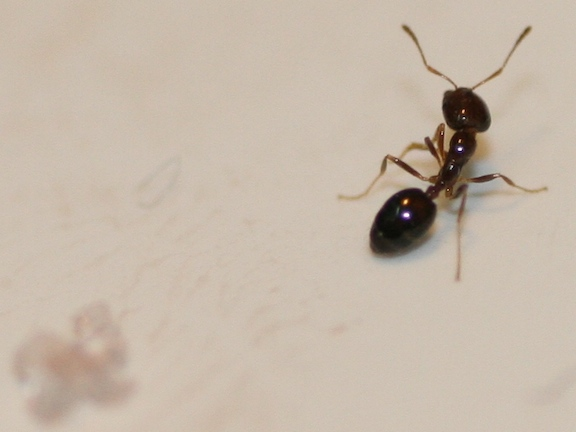Algebra

# Geometric Inequalities: Level 5 Challenges

$\large S=\left| \sqrt { { x }^{ 2 }+4x+5 } -\sqrt { { x }^{ 2 }+2x+5 } \right| \quad \forall x \in \mathbb R$

For $S$ as given above, find the maximum value of $S^8$.

Notation: $| \cdot |$ denotes the absolute value function.

Positive reals $x,y,z\ge \dfrac{\sqrt{3}}{3}$ satisfy the condition $xyz+x+y-z=0$. If $kxyz-xy-yz-zx\ge 1$ is always true, the the minimum value of $k$ can be expressed as $\dfrac{a\sqrt{b}}{c}$ for positive integers $a,b,c$ with $a,c$ coprime and $b$ square-free.

What is $a+b+c$?A 12 feet high room is 40 feet long and 10 feet wide. Brilli the ant is standing in the middle of one of the walls (which is 10 by 12 feet), such that it is 1 foot above the floor, and is equidistant from the other 2 edges of that wall.

In the middle of the wall opposite to Brilli's, rests a sugar crystal, 1 foot below the ceiling. The minimum distance Brilli needs to cover to reach the sugar crystal, assuming that Brilli can walk anywhere inside the room, is $x$. Determine the value of $x^2$.

###### Image credit: Flickr Snap

What is the smallest value (round off to 1 decimal point) that

$\sqrt{49+a^2-7\sqrt2a}+\sqrt{a^2+b^2-\sqrt2ab}+\sqrt{50+b^2-10b}$

can have for positive real numbers $a$ and $b$?

Find the square of the minimum value of $\sqrt{9+x^{2}}+\sqrt{(4-x)^{2}+(3-y)^{2}}+\sqrt{4+y^{2}}$ as $x$ and $y$ range over all real numbers.

×